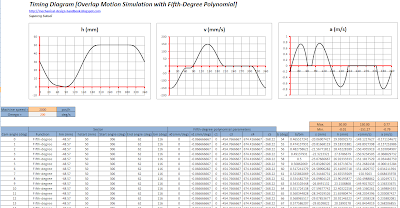Improve math skills of your kids - Learn step-by-step arithmetic from Math games

Math: Unknown - Step-by-step math calculation game for iOS.

Math: Unknown is much more than a math game. It is a step-by-step math calculation game which will teach users how to calculate in the correct order rather than just asking only the final calculated results.

The app consists of four basic arithmetic operations which are addition, subtraction, multiplication and division. In order to get started, users who are new to arithmetic can learn from animated calculation guides showing step-by-step procedures of solving each type of operation. It is also helpful for experienced users as a quick reference.

Generally, addition and subtraction may be difficult for users who just start learning math especially when questions require carrying or borrowing (also called regrouping). The app helps users to visualize the process of carrying and borrowing in the way it will be done on paper. Once users understand how these operations work, they are ready to learn multiplication and division.

For most students, division is considered as the most difficult arithmetic operation to solve. It is a common area of struggle since it requires prior knowledge of both multiplication and subtraction. To help users understand division, the app uses long division to teach all calculation procedures. Relevant multiplication table will be shown beside the question. Users will have to pick a number from the table which go into the dividend. Multiplication of selected number and divisor is automatically calculated, but the users have to do subtraction and drop down the next digit themselves. Learning whole calculation processes will make them master it in no time.

Math: Unknown is a helpful app for students who seriously want to improve arithmetic calculation skills.

Polynomial Cam Function (Fifth-degree polynomial Example) - Part 4

In the post [Polynomial Cam Function (Fifth-degree polynomial characteristics) - Part 3], we know the characteristics of Fifth-degree polynomial cam profile. In this post we will see the example of using Fifth-degree polynomial together with Linear cam functions to improve the movement of mechanism. We can use the same example as what we did in the post [Timing Diagram (Part 4 - Timing Diagrams Comparison using Motion Simulation in Microsoft Excel)]. The original maximum acceleration of the die for that case without any overlap motion was 4.154 m/s2. But we did the improvement using cycloid cam curve and the maximum acceleration reduced to 0.804 m/s2. That was a big improvement on the acceleration of the mechanism. This time we can find that using Fifth-degree (3-4-5) polynomial cam function can also considerably reduce the maximum acceleration of the mechanism in the same level as cycloid cam profile. But the comparison between cycloid and fifth-degree polynomial for this case is not that significant. This is just to show how to use it. And please note that it doesn't mean that lowest acceleration is best movement for this kind of application. This is just only the example of using overlapping motion with fifth-degree polynomial cam profile.

Our constraints for this motion is still the same as previous example, the total displacement is 50 mm and the die has to move inside the hole for 30 mm. The die has to be away from the indexing mill surface about 1 mm before indexing mill really moves.

Please note that the velocity and acceleration functions derived in previous post has the unit of mm/rad and mm/rad2 respectively. To change the angular domain to time domain, we have to multiply velocity function and acceleration function by omega and omega2 respectively. Where omega is the angular velocity of cam shaft in rad/s. This is when v0 and v1 are in mm/rad and bm in deg. But normally it's easier to use v0 and v1 in mm/deg and bm in deg. So the unit of omega should be deg/s.

We can work out in more details and get the following displacement, velocity and acceleration profiles as shown in the following graphs.

Sector   Function        Start Angle   End Angle    h1         h2
A-B     Linear             62                77                1.43       0.43
B-C     Fifth-degree     77                206              0.43        50
C-D     Dwell             206               306                50         50
D-A     Fifth-degree    306                62                 50        1.43

We know that fifth-degree (3-4-5) polynomial cam function needs 2 extra parameters i.e. v0 and v1 to define the full curve of each sector. For this example, the linear cam function is used between 2 fifth-degree polynomial sectors. The slope of linear cam function then defines values of v1 and v0 of first and second fifth-degree polynomial cam curves respectively.

The slope of linear cam function can be calculated from (0.43-1.43)/(77 -62) = -0.0667. And this value is the value of v1 of sector D-A and is the value of v0 of sector B-C. This is to make sure that there is no discontinuity at the connecting point between velocity cam profiles.

Remark about displacement profile: The fifth-degree polynomial displacement function derived previously has zero displacement as starting point and end with total lift of hm. To connect it with other curve, we must add another value called hstart in order to avoid discontinuity in displacement cam curve. Find more details in the Excel spreadsheet example file.

A simple excel spreadsheet is developed as shown below to calculate for displacement, velocity and acceleration over the entire cycle of movement. Graphing function in Microsoft Excel makes it easy to visualize any discontinuity that may have. We can also simply trace all the displacement, velocity and acceleration over all sectors. With excel graph, we can easily find the sector that creates highest acceleration (absolute value of both positive and negative acceleration) and can modify the timing diagram until we get the best result.In the excel spreadsheet, you can see motion simulation to compare between movement using cycloid and fifth-degree polynomial.

FREE DOWNLOAD EXCEL FILE OF TIMING DIAGRAM OVERLAP MOTION WITH FIFTH-DEGREE POLYNOMIAL CAM FUNCTION.

Extract the zip file with password: mechanical-design-handbook.blogspot.com